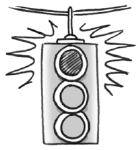### Home > GC > Chapter 5 > Lesson 5.3.3 > Problem5-91

5-91.Jerry was trying to use a flowchart to describe how his friend Marcy feels about Whizzbangs candy. Examine his flowchart below.

1. How do you know Jerry’s flowchart is incorrect?

The fact that Marcy likes chocolate does not cause Whizzbangs to be $100\%$ chocolate.
How should the arrows be changed?

2. Make a flowchart on your paper with the same three ovals, but with arrows drawn in so the flowchart makes sense. Explain why your flowchart makes more sense that the one below.

 $\enclose{circle}{\; \\ \quad \;\; \text{Marcy likes} \quad \\ \qquad\text{chocolate}\\}$ $\$$\huge \leftarrow$ Bubble 1 on the right has an arrow pointing to bubble 2 on the left $\enclose{circle}{\; \\ \quad \, \text{Marcy likes} \\ \quad \text{Whizzbangs} \quad\\}$ Bubble 2 on the left has an arrow pointing down to bubble 3$\huge \searrow$ $\huge \nearrow$  Bubble 3 on the bottom has an arrow pointing up at bubble 1. $\enclose{circle}{\; \\ \quad \;\; \text{Whizzbangs are} \quad \\ \quad \; \; \,\text{100% chocolate}\\}$
 $\enclose{circle}[mathcolor=red] {\color{black}{\; \\ \quad \;\; \text{Marcy likes} \quad \\ \qquad\text{chocolate.}\\}}$ $\enclose{circle}[mathcolor=red] {\color{black}{\; \\ \quad \;\; \text{Whizzbangs are} \quad \\ \quad\; \; \text{100% chocolate.}\\}}$ Bubble 2 has an arrow pointing down at bubble 3.$\huge \searrow$ $\huge \swarrow$ Bubble 1 has an arrow pointing down at bubble 3. $\enclose{circle}[mathcolor=red] {\color{black}{\; \\ \quad \; \text{Marcy likes} \\ \quad \text{Whizzbangs.} \quad \\}}$